# Solar Resource Analysis without Uncertainty

This webpage describes how to make a resource analysis of solar power, meaning an estimate of how much solar power can be produced in a given time period in a given location.  The resource analysis explains how to go to various websites and collect data on solar energy that hits a panel (in-plane energy, point of access energy).  With this information and estimates of the performance ratio you can compute net solar energy very easily. As such, factors that go into the measuring the performance ratio and the definition of the performance ratio is addressed.  Terminology and calculation of things such as solar yield, performance ratios and temperature coefficients are also addressed. A few files are used in the resource analysis.  The first file is write-up of the resource estimation process. that works through acquiring data, performance ratios and temperature coefficients.  The second file is and excel file that extracts data on the cost of solar panels and contains a time series evaluation of solar costs.  The third file is a set of power point slides that works through the solar resource estimates.

Solar resource analysis is fundamental to the analysis of a solar project for many reasons. This typical project finance diagram illustrates how solar power resource is not mitigated by other contracts (even though some people are trying make products that guarantee the production).## Gross Solar Resource Estimates Using Public Websites

Solar power is different from other technologies in that you can perform a whole lot of resource analysis from the internet on specific projects in different locations.  You can get estimates of solar resources; you can get historic data for computing P90, P99 etc.; you can solar capital get cost data; and you can find data for evaluation of the cost of capital. Some websites where you can find solar resource data include Retscreen, JRC, PVWATT and NREL (I think the top link is the best). To get the Retscreen data you need to download the retrscreen tool.

### http://pvwatts.nrel.gov/

By far the best website is the one from the EU (see, Brexit, the EU is good). I will walk through a few very simple ways in which you can assess the resource analysis without being a fancy engineer.  I compare the energy yield that comes from a project in Norther Nigeria with a project in Scotland. The files and videos below walk through analysis of the solar resource, meaning the amount of solar energy that is available at different places in the globe. An file the works through computing solar production from various sources can be downloaded by pressing the button below.

When opening this website, you get a nice map and then you can press on the area to find the resource.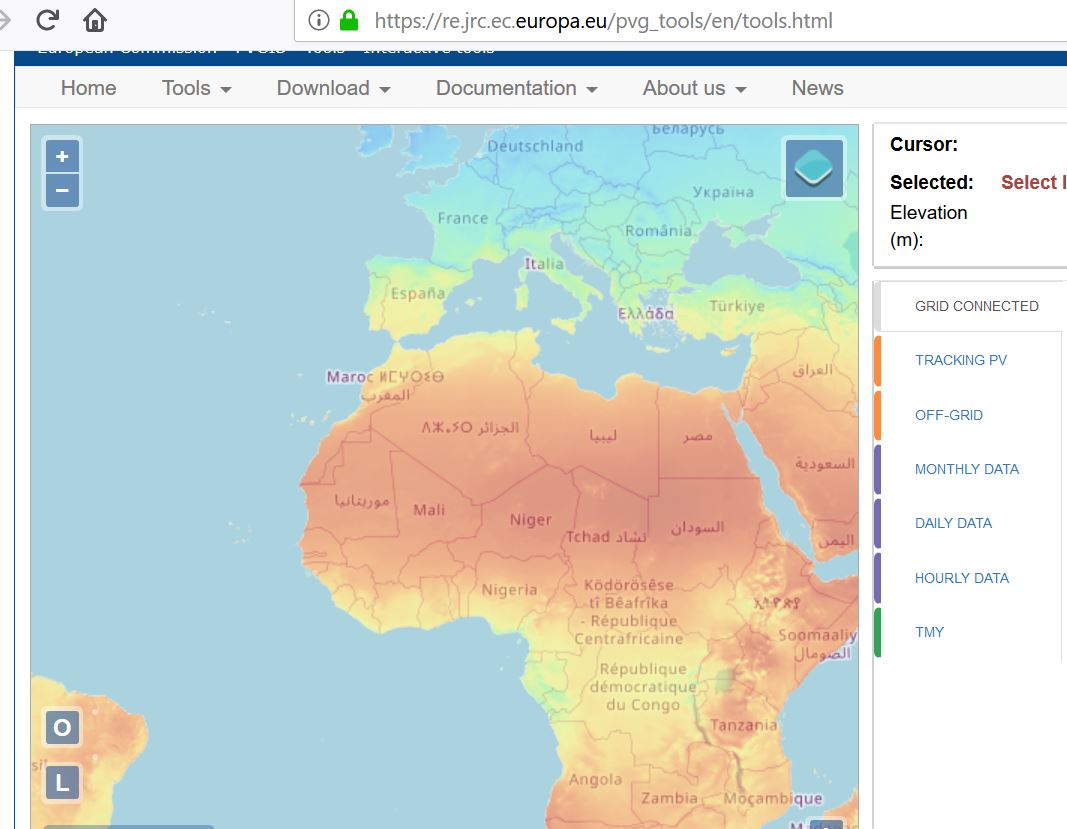In solar, all costs including financing costs and operation costs are fixed. If you have twice as much output the cost is halved. Imagine two factories with the same fixed cost and no variable cost. One factory produces half as much as the other. The factory with lower production must receive twice the price.

So, lets do a very simple comparison between Scotland and Northern Nigeria. Concentrate on the in-plane radiation that hits the panels. Yield in kWh/m2 divided by 8760 gives you the capacity factor: 2780/8760 is 31.7%. You won’t find much better places than this.  Note that this includes single axis tracking.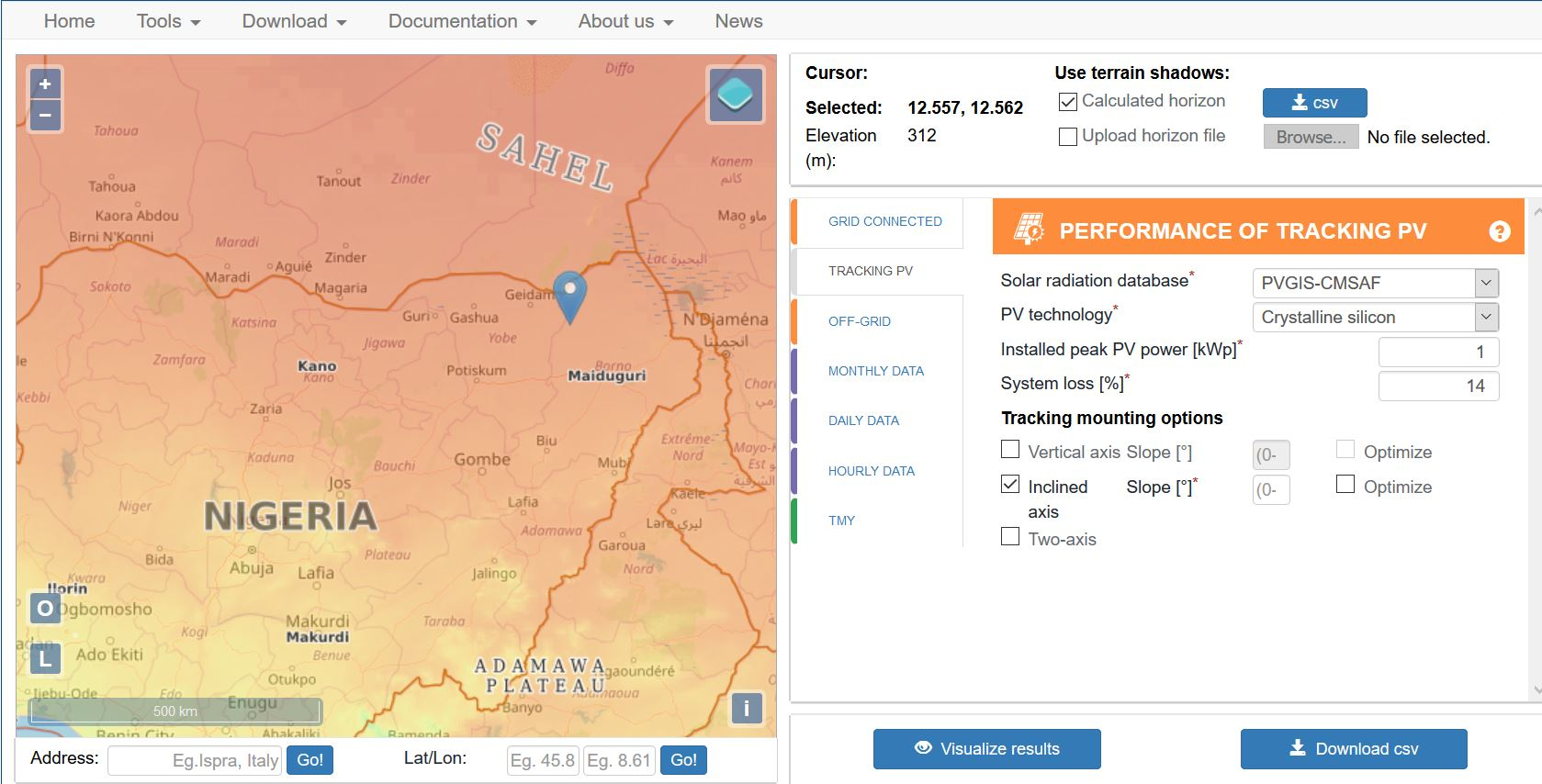Now, with tracking, the yield per kWh on the plane of the solar panel is only 1,010 kWh/m2. This produces a capacity factor of 11.52% (1010/8760).The data is not perfect and there are alternative estimates (this should be a big deal in the P90/P50 analysis with uncertainty.  I think the one we are talking about with this EU website is the solar GIS.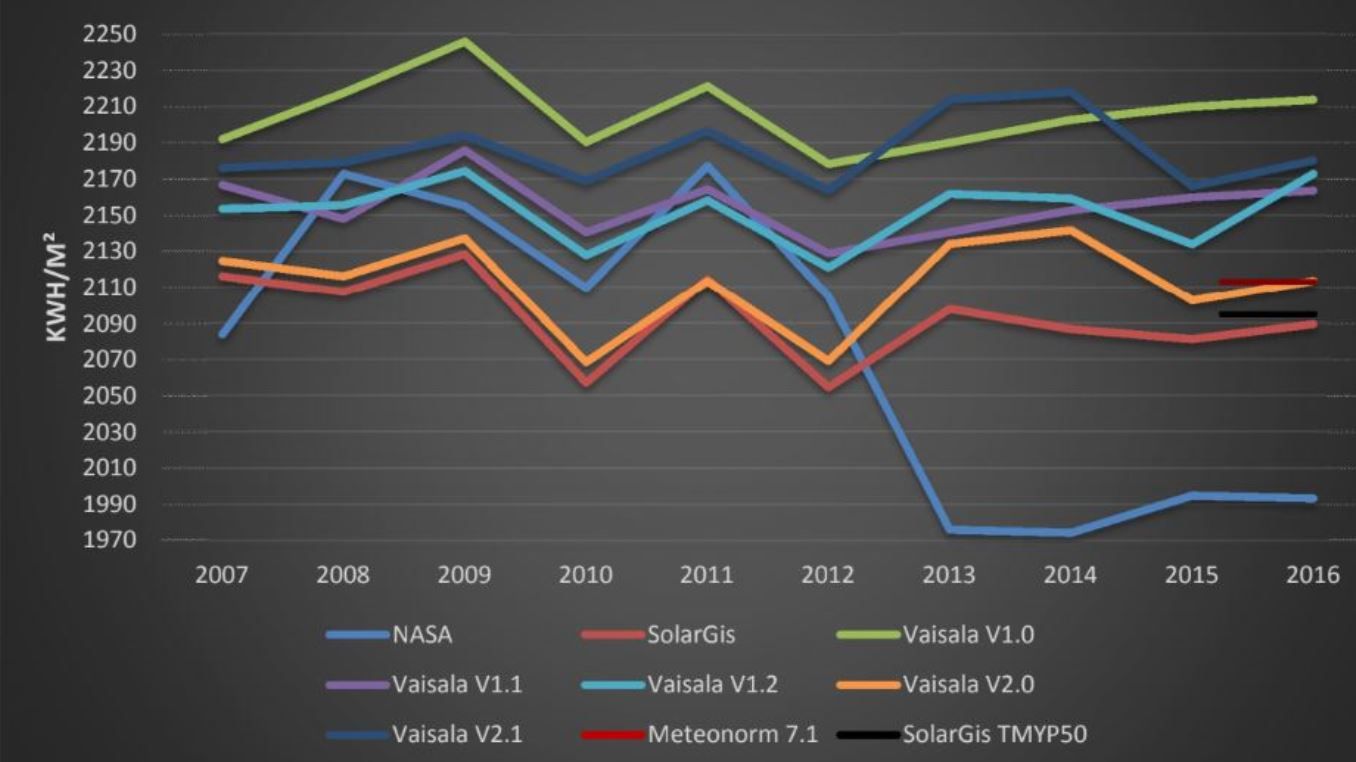The capacity of a panel is defined by something called kWp or MWp where the p represents peak and the measured instantaneous capacity is measured at a term called Standard Testing Conditions or STC. Standard testing conditions are how much power would be produced by a panel if there was solar energy touching the panel of 1,000 W/meter squared (measured with a flash test) and the temperature is 25 degrees Celsius.

If the average amount of energy over the year is 150 W/meter squared and the temperature is always 25 degrees and there are no losses from wiring, snow, shading, dust etc., then the capacity factor would be 15%.  This is simply the average production divided by the maximum production.  It is 150/1000, where the 150 is the average production per hour over the entire year and the maximum production is the 1,000 Watt defined as part of the STC.

In this case with an average production of 150 W/meter squared, you can calculate the yield.  The yield is often uses in the industry and has the same information as the capacity factor. In this case, the yield would be 150 x 8760/1,000 or 1,314 kWh/kW. The units for yield are really hours and the yield divided by the hours in a year — 8760 — is the capacity factor.  These simple equations are summarised below:

Capacity Factor = Average Production (kWh per year/8760)/Capacity (kWp)

Yield = Total Production per Year/Capacity (kWp)

If there were no clouds or dust and the sun did not have sun spots, then the amount of solar power (extra terrestrial that sounds like science fiction) could be computed direct from longitude and latitude. But clouds and other things complicate the calculation. These conditions can be measured with satellites that are going around the globe and measuring all sorts of atmospheric conditions. Because of all of this, you can click on a google map type picture and then get the amount of solar power per kW per meter squared in different places.  The capacity factor calculation is illustrated in the screen shot below.  In this case the capacity is 1,000 Wp.  In the AC System column, the total generation of 1462 kWh can be divided by 8760 to come up with average production of  166.89 Wh.  The 166.89 divided by the capacity of 1000 Wp gives the capacity factor of 16.9%.  The capacity factor at the plane of the solar panel can also be computed. This is 1969.88 x 1000/8760 to arrive at the average irradiation.  This number is again divided by 1000.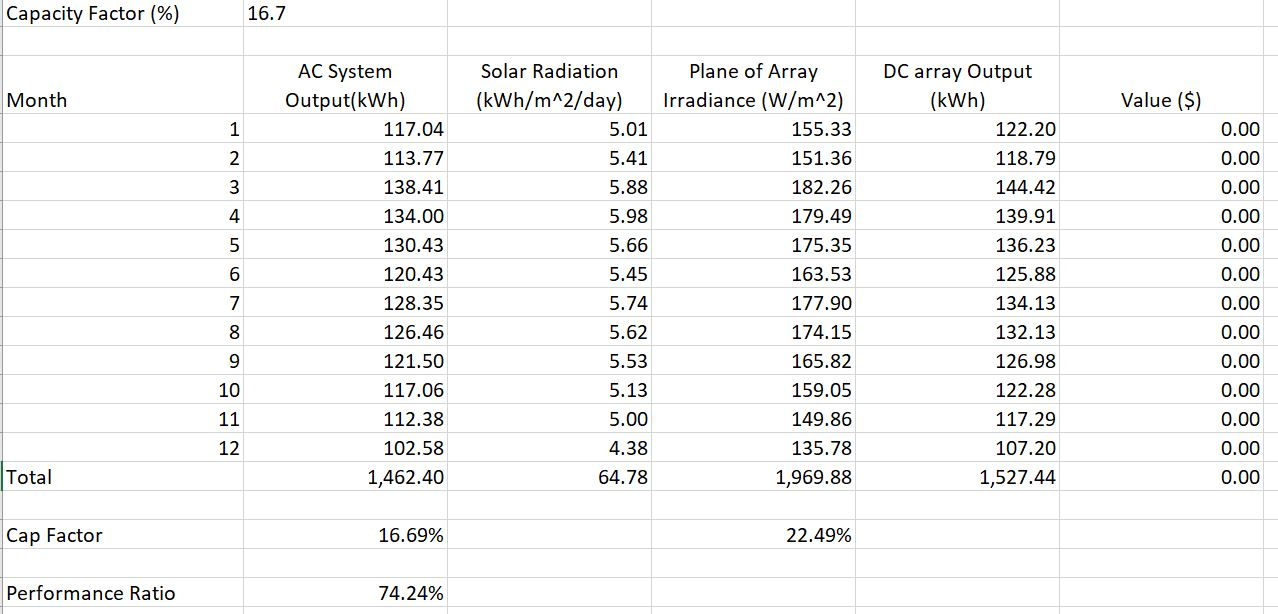The actual production of solar power depends on the performance ratio as well as the amount of sunlight.  The performance ratio reflects characteristics of the solar panel and other equipment including the reaction to temperature, losses and other factors. The screenshot below (taken from the above file) illustrates that different sources of solar data and different estimates of the performance ratio can result in large differences in estimated solar production.  The performance ratio is the final capacity factor divided by the point of access capacity factor that comes from the solar analysis and is independent of the solar panel type.The second screenshot demonstrates the importance of the performance ratio and shows the DSCR required to cover uncertainty in the performance estimate.  The DSCR is computed as 1/(1 – Percent Difference).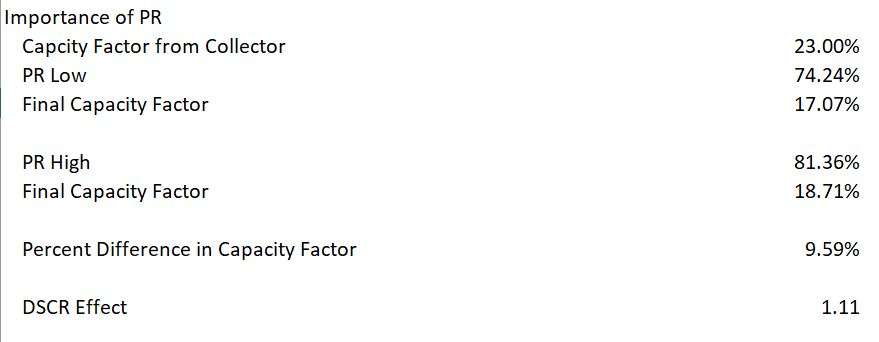## Interpreting Solar Irradiation From PVSYST and Other Sources

The discussion below shows how to take various sources of solar power and then adjusts them by the performance ratio (temperature, losses etc.).  With the performance ratio you can compute the solar yield in a crude but reasonable manner.

In this section I explain how you can compute the performance ratio using different sources.  The first example is the EU website which reports Em as the final production that is sent to the grid.  Hm is the solar production received by the module.  The screenshot demonstrates how the performance ratio is the final production capacity factor divided by the energy that hits the modules (panels).  This is again taken from the solar yield file that is available by clicking on the button in the last section.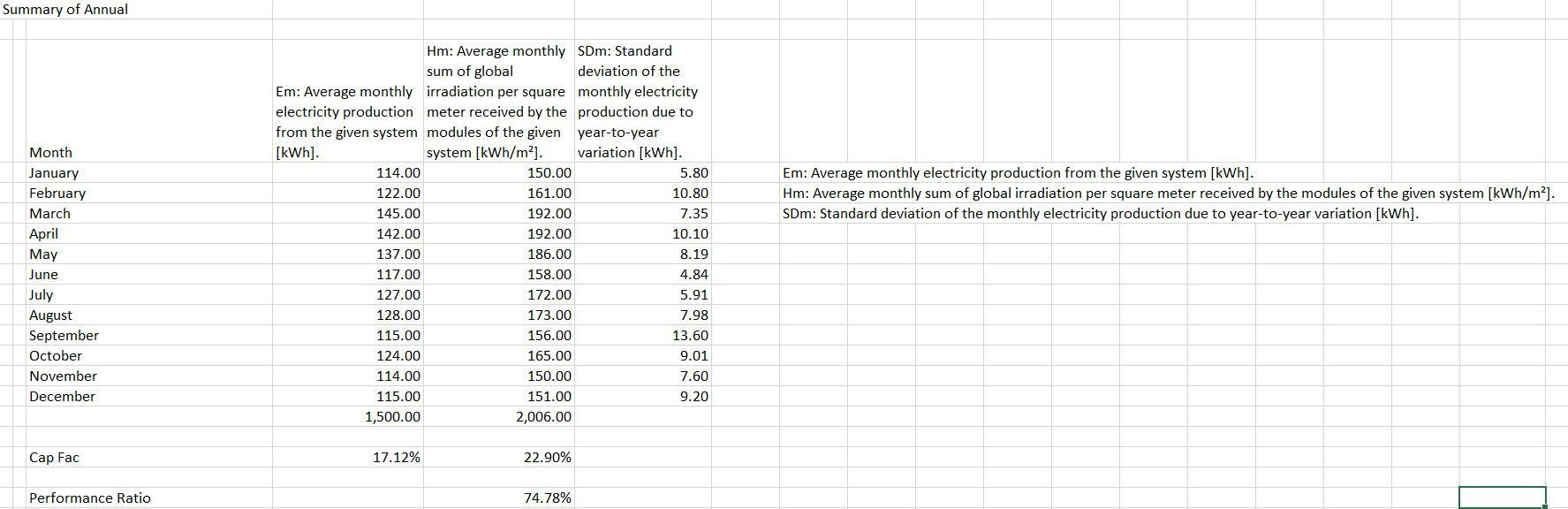The second screenshot shows outputs produced by the PVSYST model.  There are some names that are tricky to interpret.  The units are different for the GlobInc column and the E_Grid column.  But don’t worry.  Just compute the capacity factor and understand which column reflects the amount of solar production that hits the panel.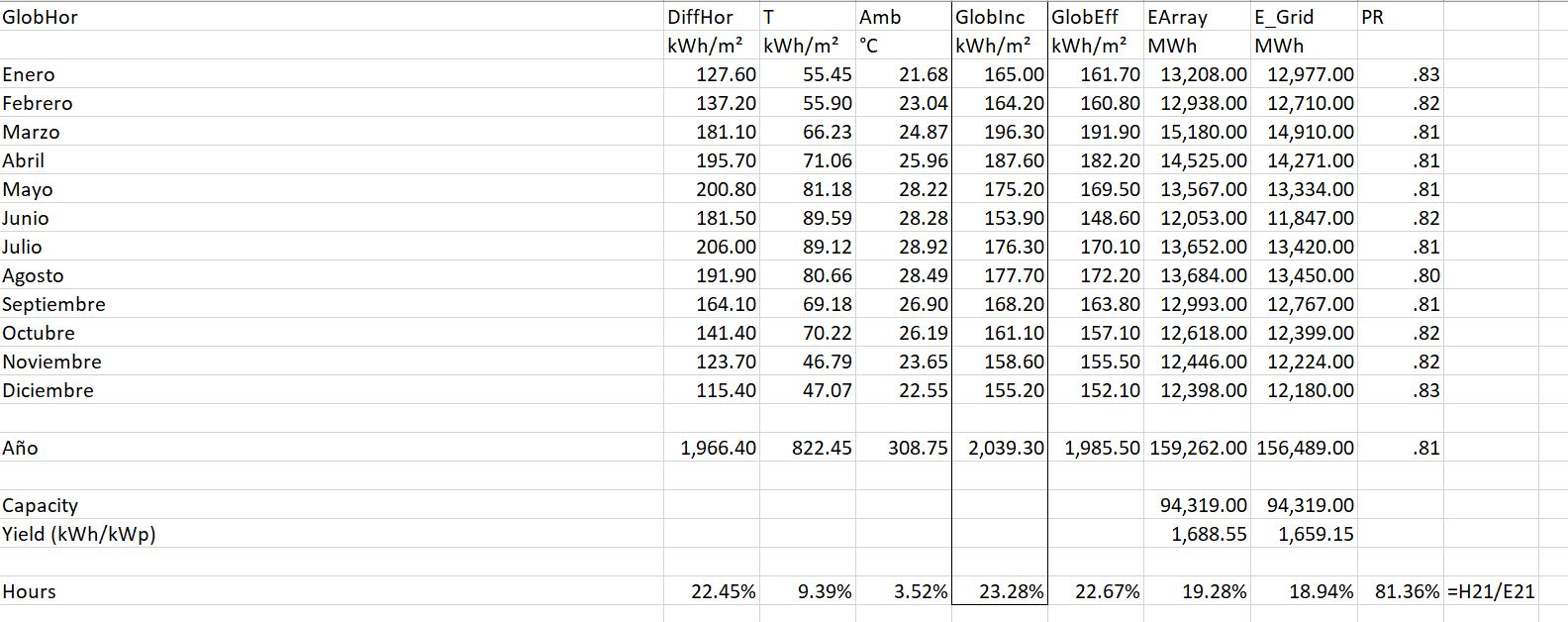The third screenshot shows the output from Heilioscope.  As with the PVSYST you should find the radiation that hits the collector and the energy to the grid.  You should get them in the same units by computing the capacity factor.  Then you can divide the final energy to the grid by the collector capacity factor (by understanding STC and 1,000 W/m2).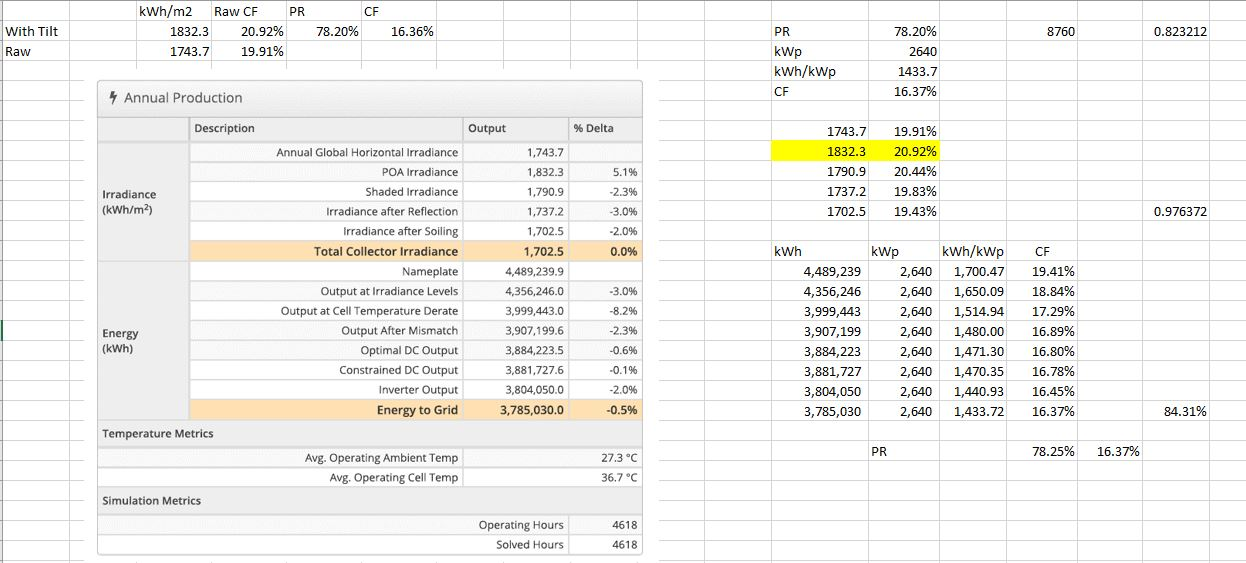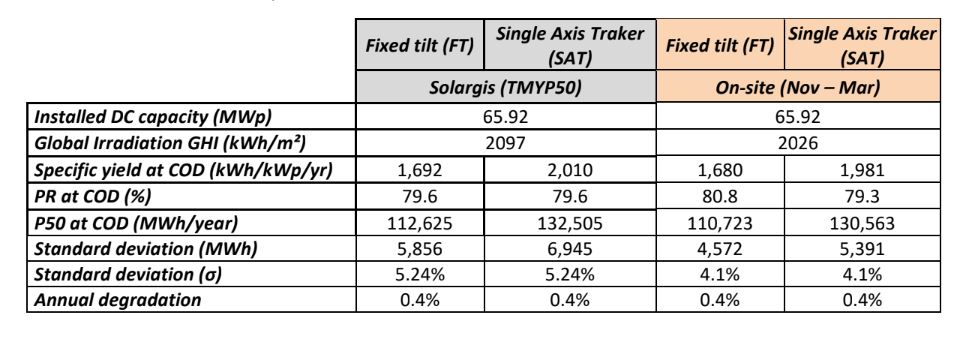## Videos Describing How to Evaluate Solar Production

I have made a couple of videos below that describe how to use websites in developing resource estimates of solar power.  The videos include explanation of how to download data from PDF files and evaluate the implied performance ratio and the capacity factor in different places.

• Solar Yield Analysis.xlsm
• Performance Ratio Analysis.xlsx
• Hourly Data from Weather Stations:

http://rredc.nrel.gov/solar/old_data/nsrdb/1991-2010/NCDCStationData/

There are a lot of solar pages related to the files and the methods described below.

## Files associated with Lesson Set 1: Solar Resource Analysis

The first step in the lesson set is evaluating solar resource. The files first set of files involve putting solar data together in a file. The basis for computing P99 etc is the NREL website and download the zipped file for a location (sorry that this is only U.S. data). The first set are files work with detailed raw data from NREL that has hourly data from 1991-2010. If you try this, go to a file and then run the MOVE macro and put each of the in a file. You can do this by opening the template file below and pressing SHIFT, CNTL, X. In addition to files that work through the solar data I have included a database of actual solar projects that is called the solar database. This database allows you to evaluate P90, P99 etc. on the basis of actual data.

Get Detailed Solar Data.xlsm

## Solar Project Finance Model

The second lesson set involves a step-by-step project finance analysis of a solar project. Building a solar project finance model is not very different than some of the other lesson sets that are described in the page name “Project Finance Exercises”. The lesson set therefore focuses on incorporating solar resource analysis into a project finance model.

These Files are Available on the Library. Get them by e-mailing edwardbodmer@gmail.com

• Solar Sensitivity Analysis.xlsm
• Assumptions.xlsx
• Solar Pre-tax Cash Flow.xlsm
• Solar Project Finance Model – Cash Flow Equations.xlsm
• Solar Project Finance Model – Basic Financing Equations.xlsm
• Solar Plant Characteristics.xlsm
• Solar Project Finance Set-up.xlsm
• Solar Project with Financing Inputs.xlsm
• Solar Project Finance Model – Basic Financing Equations.xlsm
• Solar Project Finance Model – Cash Flow Equations.xlsm

### Levelised Cost of Electricity and Currency Analysis in Solar Project Finance

• Currencies in Solar Model.xlsm
• Solar LCOE Model.xlsm
• 23. Carrying Charge Analysis Revised.xlsm
• Solar Annual Model.xlsm
• Solar Sensitivity Analysis.xlsm
• Solar Model Version 3.xlsm

In the discussion below I try to walk you through how to find the data and then compute the levelised cost of electricity from solar power in different places. While the levelised cot of electricity can be a controversial statistic, I argue that if the total cost of producing power from solar energy covering all of the fixed costs is less than the marginal cost of electricity, then solar is clearly economic.  To be more precise, if the real LCOE (that is, in today’s currency) for solar is less than the variable cost of producing power from other sources, then it is better to throw in a bunch of panels and reduce energy cost.

If you go to the page that describes LCOE, you can see the relatively simple formulas that can be used to compute LCOE.  The formulas require capital cost, capacity factor, O&M cost and the carrying charge rate.  This page explains two of the key factors in solar analysis — the capital cost of solar and the capacity factor.  The process of computing the solar LCOE is described in detail in the attached using the First Solar Case Study (It is a bit long so I am told nobody will read it).